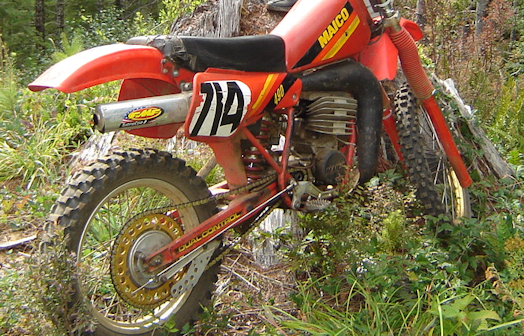Sunday, December 3, 2023 | TSTMThe transmission is a collection of gears that change the revolution ratio of the drive shaft which rotates the rear tire. The input to this system comes from the clutch which is rotated by the crank shaft via a gear driven system or a primary chain. This section I will provide an example of how gears work and this example will be the 1982 Maico 490 GS transmission.

The Maico 490 GS has three shafts in the transmission. The "MainShaft" which is the power input, the main shaft is attached to the clutch and rotates the transmission gears. The "Layshaft" which is the shaft that is rotated by the main shaft and finally the drive shaft which is rotated by the lay shaft. The full sequence of events that occur in the Maico 490 GS are as follows.

## Horse PowerHorsepower is generally used to relate how powerful an engine is in relation to the number of horses it would take to equal it's power and is generally stated to be "33,000 foot pounds of work per minute". The horse power output of an engine varies depending on the torque and the RPM of the crankshaft. The formula for computing horse power is HP = ((Torque)*(RPM))/5252. The 5252 is a constant that represents 1 horse power, if Torque (foot pounds) * RPM is 5252 then you have 1 horse power generated as output. This constant is calculated by taking the amount of foot pounds in 1 horse power (33,000) and converting it into radians per revolution (2*PI) so 5252 = 33000/(2*PI). The general equation is "Power = (Force * Distance) / Time" which in this case force is torque in foot pounds, distance is the revolutions per minute of the crank shaft, time is calculated as 1 horsepower in a single revolution and power is in units of "horse power" or 33,000 foot pounds.

Let's take a look at severals specifications for the KTM 495's maximum power output.